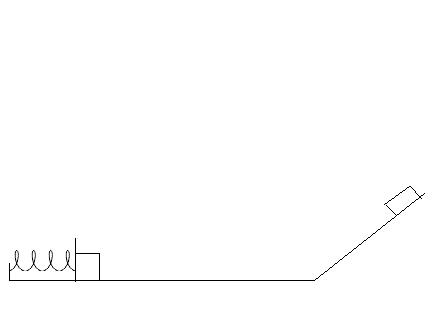# A mass of 8 kg is compressed into a spring on a horizontal surface. It is compressed into the...

## Question:

A mass of 8 kg is compressed into a spring on a horizontal surface. It is compressed into the spring 0.88 meters and held still. The force required to do this is 524 Newtons. It is then released from rest and the mass travels over a originally frictionless, horizontal surface, but at the end of the surface there is some friction with a coefficient of kinetic friction of 0.79. It then travels up a frictionless incline with an inclination angle of 20 degrees. It travels a distance of 2 meters up the incline before it stops. What was the length, in meters, of the portion of the horizontal surface that had friction?## Energy Conservation:

Energy is a conserved quantity. It is transfered from one form to another. For example, when an object slides on a rough surface, it slows down. This is because the object loses some kinetic energy as it does work to overcome the friction presented by the rough surface. As a result, the amount of kinetic energy it loses equals the amount of work done against the friction.

We will use energy conservation principles to calculate the length of the surface with friction. Note that after the spring has been compressed, it has potential energy. This is converted to kinetic energy for the mass after it is released. When the mass passes the rough surface, it loses kinetic energy as it does work against friction. After that, the kinetic energy left will be converted to gravitational potential energy as the mass travels up the incline. Therefore, the spring potential energy minus the work done against friction minus the gravitational potential energy should equal zero.

In our case, we have a mass {eq}m \ = \ 8 \ kg {/eq}. Note that the amount of force needed to compress the spring is given by the product of the spring constant k and the displacement of the spring from equilibrium x.

{eq}524 \ N \ = \ k \ x {/eq}

Since {eq}x \ = \ 0.88 \ m {/eq},

{eq}k \ = \ \dfrac{524 \ N}{0.88 \ m} \ = \ 595.4545455 \ N/m {/eq}

The potential energy stored by the spring due to this compression is then

{eq}U_s \ = \ 0.5 \ k \ x^2 \ = \ 0.5 \ \times \ 595.4545455 \ N/m \ \times \ (0.88 \ m)^2 \ = \ 230.56 \ J {/eq}

The work done against friction is given by the product of the coefficient of kinetic friction, normal force N acting on the mass, and the length of the rough surface d. Note that the normal force equals the weight of the mass. Therefore, the work done against friction is

{eq}W \ = \ 0.79 \ \times \ 8 \ kg \ \times \ 9.81 \ m/s^2 \ \times \ d \ = \ 61.9992 \ N \ \times \ d {/eq}

The height traveled by the mass when it goes up the incline is

{eq}h \ = \ 2 \ m \ \times \ \sin 20^{\circ} \ = \ 0.6840402867 \ m {/eq}

Therefore, the gravitational potential energy it gains is

{eq}U_g \ = \ 8 \ kg \ \times \ 9.81 \ m/s^2 \ \times \ 0.6840402867 \ m \ = \ 53.6834817 \ J {/eq}

From energy conservation, we have that

{eq}\begin{align*} U_s \ - \ W \ - \ U_g \ &= \ 0\\ \\ \therefore \ W \ &= \ U_s \ - \ U_g\\ \\ 61.9992 \ N \ \times \ d \ &= \ 230.56 \ J \ - \ 53.6834817 \ J\\ \\ 61.9992 \ N \ \times \ d \ &= \ 176.8765183 \ J\\ \\ \therefore \ d \ &= \ \dfrac{176.8765183 \ J}{61.9992 \ N}\\ \\ d \ &= \ 2.852883881 \ m \end{align*} {/eq}

The length of the horizontal surface with friction was {eq}\mathbf{2.85 \ m} {/eq} correct to three significant figures.